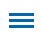Although students have been working with fractions well before sixth grade, multiplying and dividing fractions with other fractions involves more than simple computation and procedures.

An essential skill for success is the ability to rationalize if results "make sense" -- a necessary application of estimation skills. For example, when multiplying and dividing any number by a fraction, is the resulting solution less than the original multiplicand or dividend, or more? For many students, understanding that their answer is reasonable leads to a clearer understanding of the 'real world' application of these calculations.

Editor's Note: This blog is part of a series of posts from former math specialist Scott Loomis on the real world math templates he has created for Wixie and how you can use and modify them for your own awesome performance tasks. You can read the backstory on his experience and expertise below.

## School Fundraiser

The School Fundraiser template involves a bake-sale scenario that requires students to multiply or divide quantities from given recipes to bake a large or smaller number of items based on what they expect to sell. So, if 9 1/2 cups of flour are required to cook 12 dozen cookies, how much flour would you need to make 8 dozen?

Students show most, if not all, of their work as each conversion involves two or three separate pages in the Wixie template. Students begin by showing the equations they will use to solve each problem.

Instructional Concept: Apply and extend previous understandings of multiplication and division to divide fractions by fractions.

Location in Templates Library: Curriculum > Numbers and Operations > Fractions

Estimated Completion Time: 20-30 minutes

## Math Standards and Learning Goals

### Common Core Standards - Grade 6 Mathematics - The Number System

6.NS.A.1 Interpret and compute quotients of fractions, and solve word problems involving division of fractions by fractions, e.g., by using visual fraction models and equations to represent the problem.

### Virginia Standards of Learning - Mathematics - Computation & Estimation

6.5 The student will a) multiply and divide fractions and mixed numbers; b) solve single-step and multi-step practical problems involving addition, subtraction, multiplication, and division of fractions and mixed numbers.

### Texas Essential Knowledge and Skills - Mathematics - Number & Operations

TX.6.G.3. The student applies mathematical process standards to represent addition, subtraction, multiplication, and division while solving problems and justifying solutions. The student is expected to:

(B) determine, with and without computation, whether a quantity is increased or decreased when multiplied by a fraction, including values greater than or less than one;

## Wixie Skills Review

Most of the work on this template will be completed using the paintbrush tool. It is helpful to set it to its smallest size so that it can be used like a pencil.With 'work space' provided for the required calculations, students will need to toggle between pages to complete and then record their answers. This will involve the simple process of moving between pages using the storyboard.For added clarification, teachers may opt to have students add an audio recording to explain their problem-solving strategy using the Record tool.

Topics: Wixie, math, real world of math